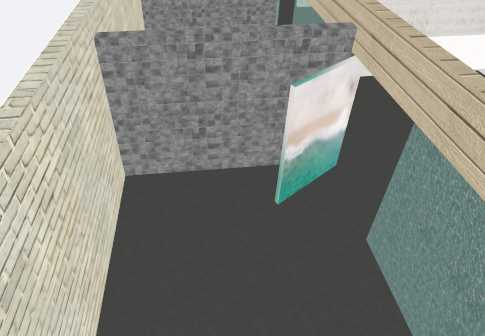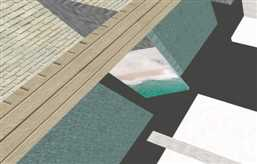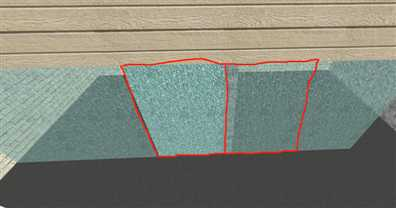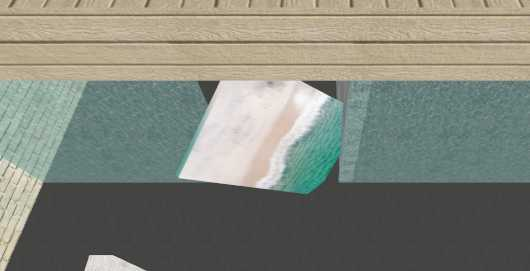## three.js中物体旋转实践之房门的打开与关闭``object.rotation.set(angleX,angleY,angleZ);````object.rotation.set(0,-Math.PI/3,0);``

①自旋转+平移

``````var rotationY = currentCube.rotation.y;
currentCube.rotation.set(0,rotationY - Math.PI/3,0);
currentCube.position.setX(currentCube.position.x + 0.4);
currentCube.position.setZ(currentCube.position.z - 0.75);``````

②添加父容器，旋转父容器ok，原理就像上图所示的那样，我们“装门”的时候，假如，门的尺寸是50X220，那么我们可以用一个100X440的盒子将这个门包起来；

①法一

``object.rotation.set(0,Math.PI / 3,0);``

②法二

``````var axis = new THREE.Vector3(0,1,0).normalize();
var angle = Math.PI / 3;
object.rotateOnAxis(axis,angle);``````③法三

``````var quaternion = new THREE.Quaternion();
quaternion.setFromAxisAngle( new THREE.Vector3( 0, 1, 0 ).normalize(), -Math.PI / 3 );
object.applyQuaternion( quaternion);``````

④法四

``````function rotateAroundWorldAxis(object, axis, radians) {
var rotWorldMatrix = new THREE.Matrix4();
rotWorldMatrix.multiply(object.matrix);
object.matrix = rotWorldMatrix;
object.rotation.setFromRotationMatrix(object.matrix);
}``````